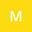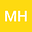A blow-up result for the wave equation with localized initial data: the scale-invariant damping and mass term with combined nonlinearities
••• Makram Hamouda,
• M.Ali Hamza
Makram Hamouda
Imam Abdulrahman Bin Faisal University
Author ProfileM.Ali Hamza
Imam Abdulrahman Bin Faisal University
Author Profile## Abstract

We are interested in this article in studying the damped wave equation with localized initial data, in the \textit{scale-invariant case} with mass term and two combined nonlinearities. More precisely, we consider the following equation: \begin{displaymath} \d (E) \hspace{1cm} u_{tt}-\Delta u+\frac{\mu}{1+t}u_t+\frac{\nu^2}{(1+t)^2}u=|u_t|^p+|u|^q, \quad \mbox{in}\ \R^N\times[0,\infty), \end{displaymath} with small initial data. Under some assumptions on the mass and damping coefficients, $\nu$ and $\mu>0$, respectively, we show that blow-up region and the lifespan bound of the solution of $(E)$ remain the same as the ones obtained in \cite{Our2} in the case of a mass-free wave equation, {\it i.e.} $(E)$ with $\nu=0$. Furthermore, using in part the computations done for $(E)$, we enhance the result in \cite{Palmieri} on the Glassey conjecture for the solution of $(E)$ with omitting the nonlinear term $|u|^q$. Indeed, the blow-up region is extended from $p \in (1, p_G(N+\sigma)]$, where $\sigma$ is given by \eqref{sigma} below, to $p \in (1, p_G(N+\mu)]$ yielding, hence, a better estimate of the lifespan when $(\mu-1)^2-4\nu^2<1$. Otherwise, the two results coincide. Finally, we may conclude that the mass term {\it has no influence} on the dynamics of $(E)$ (resp. $(E)$ without the nonlinear term $|u|^q$), and the conjecture we made in \cite{Our2} on the threshold between the blow-up and the global existence regions obtained holds true here.